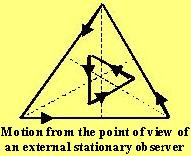## Friday, July 13, 2007

### Irodov Problem 1.12While a very mathematical and rigorous treatment of the problem is possible however, it can be solved by simply exploiting the inherent symmetry in the problem. The first step is to understand that because of the inherent symmetry in the positions and motions of the three particles, their positions at any given time will always form equilateral triangle as shown in the figure. Thus, the motion of particles will depict a shrinking equilateral triangle that is also rotating about the centroid as it shrinks. This is depicted in the figure.The problem becomes quite simple if one solves it from the point of view of an observer sitting on one of the particles. To an observer sitting on one the particles, the rotational motion of the triangle will not be present (since the triangle is always an equilateral triangle at any given point of time) and he only perceive a shrinking equilateral triangle as shown in the second figure.

To an observer located on one of the particles the components of velocities of its adjacent particle at any given point of time are
depicted in the third figure. Thus, any given particles feel thatwhile it is moving towards its adjacent particle at a speed v, the adjacent particle is moving towards it at a speed v/2. Thus the particles seem to approach each other at a constant rate of v + v/2 = 3v/2. Since the initial distance was a, the time takes to meet is given by, a/(3v/2) = 2a/3v.

#### 4 comments:

Stephen Houpt said...

I did it like this. The particles will meet in the middle. Find the original distance to the middle. Each particle always has a component of velocity toward the middle of v times the square root of 3 divided by 2. The original distance to the middle is "a" divided by the square root of 3. Divide and you've got it.

Krishna Kant Chintalapudi said...

Dear Stephen,

Indeed thats another way of solving the problem.

Since at any given time all the particles maintain the shape of an equilateral triangle (albeit a rotated version of it) due to the inherent symmetry, the component of of the particles towards the center remains the same and equal to v times square root divided by 2!

Thanks for poiting this alternative method out.

Regards
KrishnaAnonymous said...

I have one difficulty with the original solution. If we are in the frame of one of the particles we see the other particle approaching us with velocity component 3v/2. However, it also has a component of vsin60 normal to the relative position of the two. Then how do these particles converge?ta

Krishna Kant Chintalapudi said...

dear elusive self,

from the point of view of one of the particles, there is no perpendicular component. Both the particles come towards it at 3v/2 directly.

Imagine that there is an equilateral triangle shrinking and you are one of vertices and are stationary.